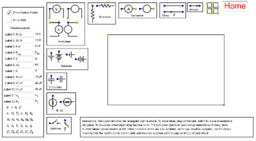Science Applets

Collection of Science applets, simulations. Physics, Chemistry Biology
•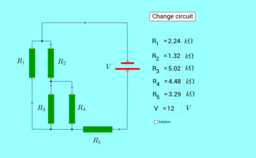Electrical circuits

•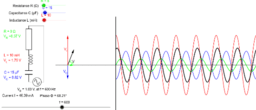L - C - R Series Circuit

••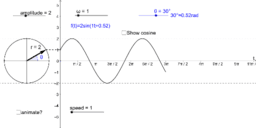Phasor Diagram and Sinusoid

•Carica del condensatore

•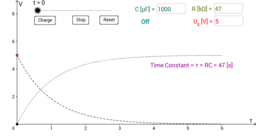RC Circuit - Charging

•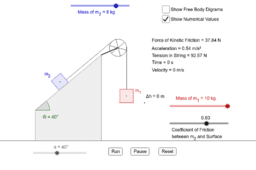Inclined Plane with Friction, Two Masses, and a Pulley

•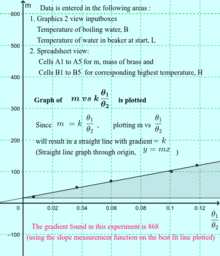Graphical Plot to Find Specific Heat Capacity of Brass

•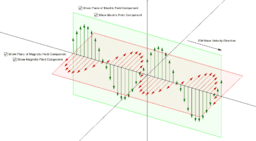Electromagnetic Waves

•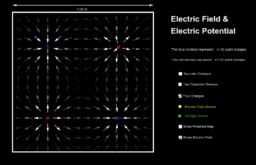Electric Field & Potential

•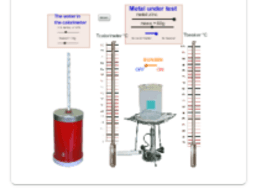Physics Applets and Simulations by Prof Luciano

•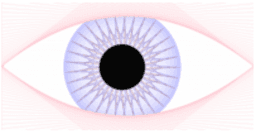Modelling Science (by Neil)

•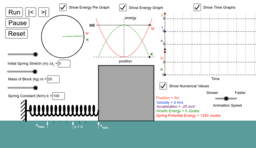Simple Harmonic Motion: Mass on a Spring

•